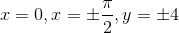# Calculus 1 : Other Curves

## Example Questions

### Example Question #1 : How To Graph Functions Of Curves

How many global extrema does the following function have?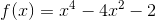Two

Impossible to determine

One

Three

Two

Explanation:

This function has only three extrema: a local maximum atand two minima at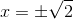(these extrema are found by finding the first derivative of the function, setting it equal to zero, and solving for x).

By evaluating any point along the four intervals defined by the extrema,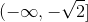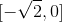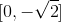, and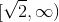, one can see that the function is decreasing on the first interval, increasing on the second, decreasing on the third and increasing on the fourth.  Therefore,is a local maximum whileare minima Furthermore, the value of the function is the same at both minima, making them global minima.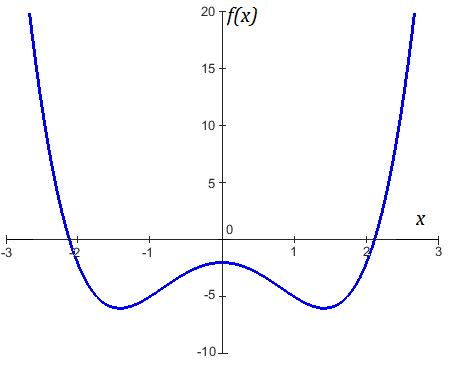### Example Question #2 : How To Graph Functions Of Curves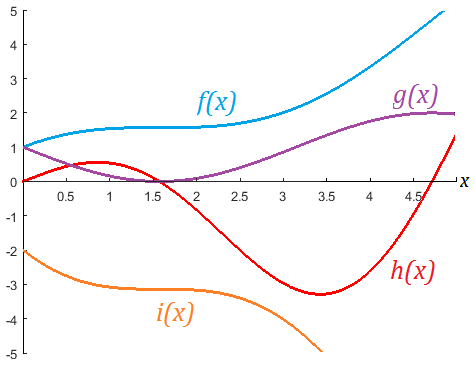For the figure above, which of the following statements is true?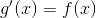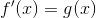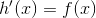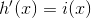Explanation:

The clearest indicators to check these answers with are (1) points where a function crosses zero compared to where another function has zero slope, and (2) where a function is increasing compared to where another function is positive.

Using the first criteria, we can see that f(x) has a slope of zero where both g(x) and h(x) cross zero, meaning f'(x) is not i(x).

Using the second criteria, we can see that f(x) is increasing on the entire interval shown, while g(x) is positive on the entire interval.  Therefore, f'(x)=g(x).

# C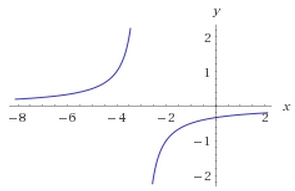D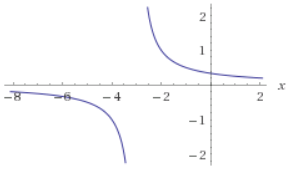For which of the graphs ofabove is the following statment true?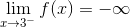D

A

B

C

A

Explanation:

The condition reads 'the limit of f of x as x approaches three from the left'.  Note that we're not looking at x as it approached negative three.  Only A and B have infinite limites at positive 3 (C and D show limits as x approaches negative 3), so the answer must be one of these.  We can see that from the left, B approaches positive infinity at x=3 from the left, while A approaches negative infinity at x=3 from the left, so the correct answer is A.

### Example Question #4 : How To Graph Functions Of Curves

On what interval is the following function decreasing?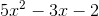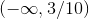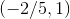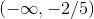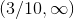Explanation:

Since this is a quadratic function with a positive first coefficient, we know it only has one local minimum and no other extrema. So all we have to do is find the x coordinate corresponding to this value and we will have one endpoint of our interval, and it will be decreasing constantly as you approach this point from the left.

Recall,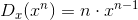So, if we take the derivative of this quadratic, we get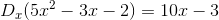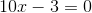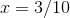So this is where our interval ends. Since there are no other local extrema, we know our interval is.

### Example Question #1 : Other Curves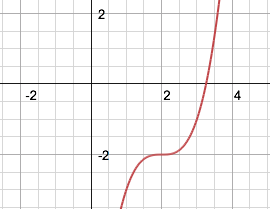Which of the following is true about the twice-differentiable functionabove?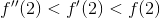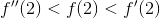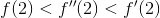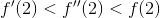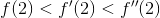Explanation:

Since the functionis increasing at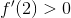, and since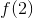is below the x-axis,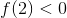.

Furthermore, there exists an inflection point at, where the concavity of functionchanges.

Thus at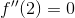.

Therefore, the correct answer is### Example Question #1 : How To Graph Functions Of Curves

Which one of the following could be the integral of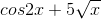?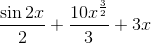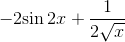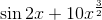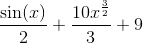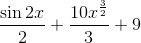Explanation:

Since the functions are added together, we can take each one seperately and add the results together.

The integral of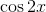is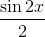since you must apply for the chain rule of the.

The integral of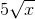will be using the power rule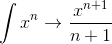,

which means it will equal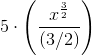, which turns into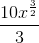,

so combining these gives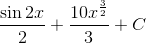as the integral, makingthe only equation that satisfies this quality.

### Example Question #1 : How To Graph Functions Of Curves

Exponential Function

What is the graph of the folloiwng function: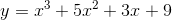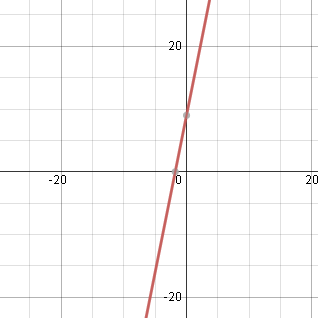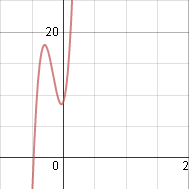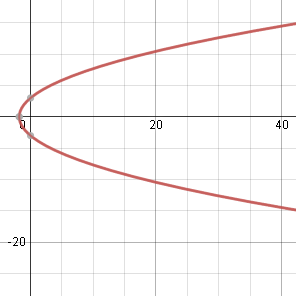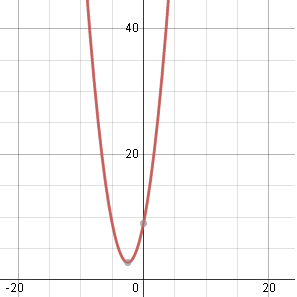None of the aboveExplanation:

Use the following values to plot the graph: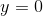,,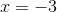,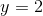### Example Question #4 : How To Graph Functions Of Curves

Trigonometric Function

Graph the folloiwng function: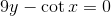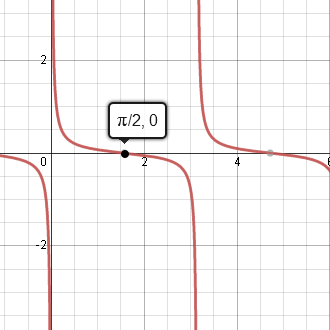None of the above